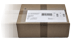# TI-30X Plus MathPrint

sales promotion
CHF 24.90CHF 28.00When you order this item your purchase will be despatched free or charge
Availability
Available from stock  Delivery within 1-3 working days!
Manufacturer
Texas Instruments
Guarantee
36 Months
GTIN
03243480105972
Dimensions
80 x 184 x 16 mm
Weight
130 g
Directions for Use
German, French, Italian

#### Items delivered

• TI-30X Plus MathPrint calculator
• Instructions (only in German, French and Italian)
• Protective cover
• Battery (1 x CR2032)

Successor to the TI-30X Plus MultiView
The TI-30X Plus MathPrint school calculator impresses with an up to 4-line high-resolution display. This guarantees easier legibility and enables additional functions e.g. the simultaneous display of two functions in the value table.
• High-resolution display with up to four lines of 16 characters
• NEW: Operating system display ensures greater exam security
• "MathPrint": Input and output in mathematical notation (as in the maths book)
• Mode menu enables easy selection of the desired type of display
• Clear and direct comparison of different calculation methods and results
• Increased functionality for statistical data analysis
• All basic scientific, trigonometrical and hyperbolic functions
• All functions required for lessons and exams for arithmetic, algebra, geometry, trigonometry, stochastics and analysis
• Prime factorisation, GCD and LCM functions
• Calculates with fractions (reduction)
• Fractions with a horizontal fraction bar and fraction function calculations (reduction, conversion of fractions/decimals/percentages; change between improper fractions and mixed numbers)
• Converts at the touch of a button: fractions, square roots and Pi into decimals and vice versa
• Easy calculation with variables and function values
• Record and store value tables and classify functions
• Displaying value tables (x, y): students can easily find a value table (x, y) for any function, either automatically or by entering specific x values.
• Two-variable statistics: input, delete, insert, add and edit individual data elements
• One- and two-variable statistics with continuous input autosave for statistical variables.
• A wide range of stochastic functions: calculates n!, means and standard deviations plus probabilities, in particular binomial and normal distributions
• Broad range of regression models (linear, non-linear and NEW: natural exponential functions (e^x))
• Calculates partial sum and partial product
• Increased functionality for statistical data analysis
• Enter and store value tables and assign any desired functions
• Basic data/list editor with three lists and list formulae
• Measures of location and dispersion, also the median and quartiles
• Calculating with complex numbers
• Summation of any Terms
• Calculates with and converts between binary, octal, decimal and hexadecimal numbers (BIN/OCT/DEC/HEX)
• Metric conversions and physical constants
• The Equation Recall function calls up previous inputs, saved values and individual statistics from the memory.
• Continuous autosave: no entry gets lost
• Memory for seven variables (x,y,z,t,a,b,c)
• Battery operation backed up by solar cells to prolong battery life
Accessories

^ top